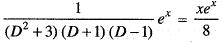Courses

# Differential Equations - 6

## 20 Questions MCQ Test Topic-wise Tests & Solved Examples for IIT JAM Mathematics | Differential Equations - 6

Description
This mock test of Differential Equations - 6 for Mathematics helps you for every Mathematics entrance exam. This contains 20 Multiple Choice Questions for Mathematics Differential Equations - 6 (mcq) to study with solutions a complete question bank. The solved questions answers in this Differential Equations - 6 quiz give you a good mix of easy questions and tough questions. Mathematics students definitely take this Differential Equations - 6 exercise for a better result in the exam. You can find other Differential Equations - 6 extra questions, long questions & short questions for Mathematics on EduRev as well by searching above.
QUESTION: 1

### The differential equation representing the family of circles touching y - axis at the origin is

Solution:

Equation of circles touching y axis is given by
( x - a2) + y2 = a2 where a is parameter implies x2 + y2 - 2ax = 0
Differentiating w.r.t. x, we get
2x + 2yy' - 2a = 0
implies a = x + yy'
So, differential equation will be
x2 +y2- 2x(x + yy1) = 0
implies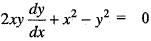So, differential equation is non-linear and of first order

QUESTION: 2

### The general solution of the differential equation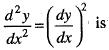Solution:

we have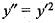on integrating, we get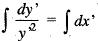implies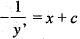implies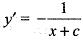implies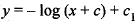implies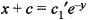implies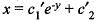where c1' is constant.

QUESTION: 3

### If e2x and xe2x are particular solutions of a second order homogeneous differential equation with constant coefficients, then the equation is

Solution:
QUESTION: 4

If ya is an integrating factor of the differential equation 2xy dx - (3x2 - y2) dy = 0, then the value of a is

Solution: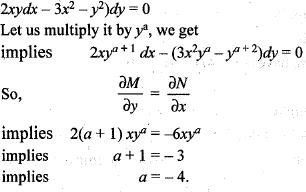QUESTION: 5

The particular integral of the following differential equation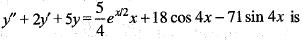Solution: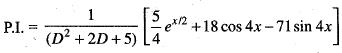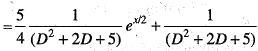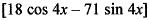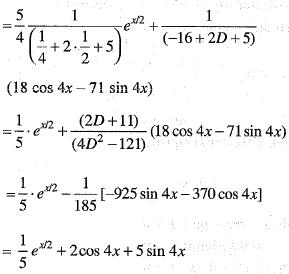QUESTION: 6

If k is a constant such that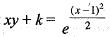satisfies the differential equation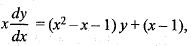then k is equal to

Solution: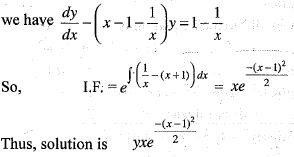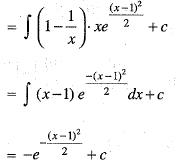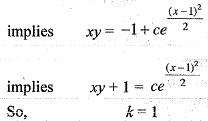QUESTION: 7

The solution y(x) of the differential equations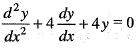satisfying the conditions y(0) = 4,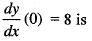Solution:

we have (D2 + 4D + 4)y = 0
implies D + 2)2 y = 0
So, solution is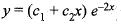but y(0) = 4 implies c1 = 4
So,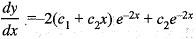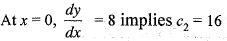So, solution is given by (16x + 4) e-2x

QUESTION: 8

The general solution of yy" - (y')2 = 0 is

Solution:

we have yy" - y'2 = 0
implies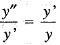on integrating, we get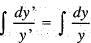implies In y' = In y + c
implies y' = c1y
Again on integrating,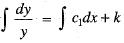implies In y = c1x + k
implies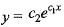QUESTION: 9

The solution of the differential equation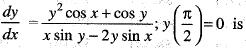Solution:

we have (y2 sin x + x cos y ) dx - ( x sin y - 2y sin x) dy = 0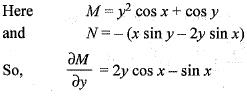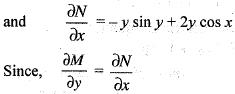So, differential equation is exact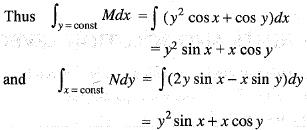So, solution is given as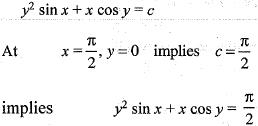QUESTION: 10

A particular integral of the differential equation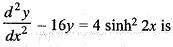Solution: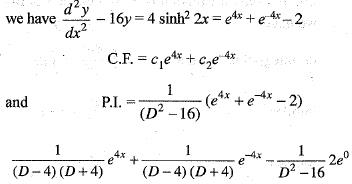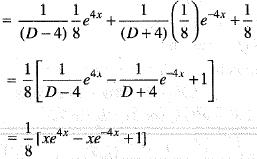QUESTION: 11

Let y1(x) and y2(x) be twice differentiable functions on a interval I satisfying the differential equations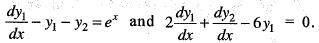Then y1(x) is

Solution:

we have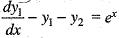implies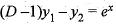...(i)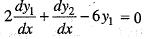implies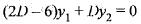...(ii)
Differentiating (i) w.r.t. x, we get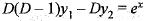...(iii)
Adding (ii) and (iii), we get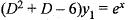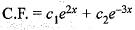and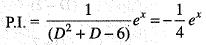So, complete solution is given as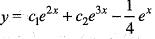QUESTION: 12

The general solution of the differential equation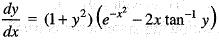is

Solution: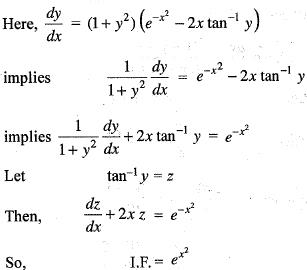Thus, solution is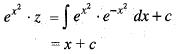implies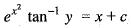QUESTION: 13

The solution of the differential equation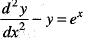satisfying y(0) = 0 and dy/dx (0) = 3/2 is

Solution:

Here, C.F. = c1 sinh x + c2 cosh x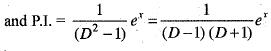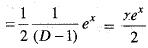So,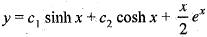but y(0) = 0 implies c2 = 0,
Thus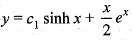implies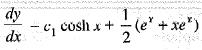At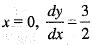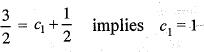So,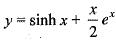QUESTION: 14

An integrating factor of the differential equation 2xy dx + (y2 - x2) dy = 0 is

Solution:

Here I.F. = 1/y2, we get
Multiplying differential equation by 1/y2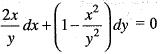Now,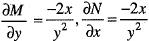Since,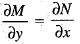So, equation becomes exact

QUESTION: 15

If y = x cos x is a solution of an nth order linear differential equation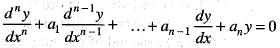with real constant coefficients, then the least possible value of n is

Solution: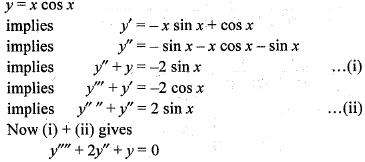So, n = 4

QUESTION: 16

Let W[y1(x), y2(x)] is the Wronskian formed for the solutions y1(x) and y2(x) of the differential equation y" + a1y' + a2y = 0. If W ≠ 0 for some x = x0 in [a, b] then

Solution:
QUESTION: 17

The general solution of y' (x + y2) = y is

Solution:

Here, y'(x +y2) = y
implies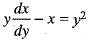implies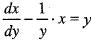So,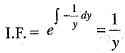Thus, solution is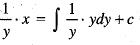implies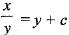implies x = cy + y2

QUESTION: 18

The general solution of y' - 2x-y is

Solution:

Here y' = 2x-y
implies 2y dy = 2x dx
implies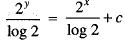implies 2x-2y = c'

QUESTION: 19

Solution of the differential equation xy' + sin 2y - x3 siny is

Solution: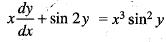implies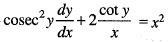Let cot y= 2
Thus,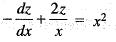implies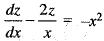So,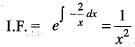Hence, solution is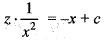implies z = cot y = -x3 + cx2

QUESTION: 20

A particular solution of the differential equation
(D4 + 2D2 - 3)y = ex is

Solution: